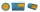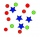# String

Divide the string length 800 cm in ratio 1: 5

a =  133.33 cm
b =  666.67 cm

### Step-by-step explanation:Did you find an error or inaccuracy? Feel free to write us. Thank you!Tips to related online calculators
Check out our ratio calculator.
Do you want to convert length units?

## Related math problems and questions:

• The stringThey cut 113 cm from the string and divided the rest in a ratio of 5: 6.5: 8: 9.5. The longest part measured 38 cm. Find the original length of the string.
• Ratio6 numbers are in the ratio 1:5:1:5:5:5. Their sum is 242. What are the numbers?
• Divide moneyDivide 1200 USD at a ratio of 1:2:3:4:5:6:9:10
• DonutsFind how many donuts each student will receive if you share 126 donuts in a ratio of 1:5:8
• RatiosReduce the numbers: 50 in a 1:2 ratio 111 at a ratio of 2:3 70 at 10:50 560 at a ratio of 3:8
• AgesJohn, Teresa, Daniel and Paul have summary 56 years. Their ages are in a ratio of 1:2:5:6. Determine how many years have each of them.
• Map 2At what scale is made map if the distance 8.2 km corresponds on the map segment 5 cm long?
• Divide in ratioLine segment AB 12 cm long divide in a ratio of 5: 3. How long are the individual parts?
• Moneys in triple ratioMilan, John and Lili have a total 344 euros. Their amounts are in the ratio 1:2:5. Determine how much each of them has?
• Similar trianglesThe triangles ABC and XYZ are similar. Find the missing lengths of the sides of the triangles. a) a = 5 cm b = 8 cm x = 7.5 cm z = 9 cm b) a = 9 cm c = 12 cm y = 10 cm z = 8 cm c) b = 4 cm c = 8 cm x = 4.5 cm z = 6 cm
• On the mapA line 1.5 cm long corresponds to a line 3 cm long on the map. What is the scale of the map?
• Scale of the mapDetermine the map's scale, which is the actual distance of 120 km l represented by a segment long 6 cm.
• Ratio - rectangleThe rectangle has dimensions 6 cm and 9 cm. How many times increases area and its perimeter, when its dimensions increase in the ratio 5:3?
• ŽSRCalculate fixed annual personnel costs of operating monorail line 118 km long if every 5 km is a station, which serves three people - one dispatcher and two switchmen in 4-shift operation. Consider the average salary of the employee 885 €.
• Divide 5Divide 288 in the following ratio 3 : 4 : 5
• Wood cuboidWhat is the weight of the wood cuboid 15 cm, 20 cm, 3 m if 1 m3 wood weighs 800 kg?
• CuboidThe sum of the lengths of the three edges of the cuboid that originate from one vertex is 210 cm. Edge length ratio is 7: 5: 3. Calculate the length of the edges.